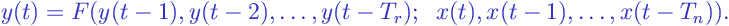# Recursive Filter

Recursive Filter:

In recursive filters , the outputat the momentis a function of the output valuesat the previous moments and, probably, of the input values.

A major advantage of recursive filters over nonrecursive filters is that they are computationally simpler. For example, for any recursive linear filter , there is a functionally equivalent non-recursive linear filter. But the non-recursive variant may be characterized by an infinitely large window size and, hence, be difficult for practical implementation. (See, for example, explanations on the exponential filter ).

Mathematically, a recursive filter may be described by the following general expression:where

•is an arbitrary function of the previous output valuesand input values, linear or nonlinear.

Some examples of recursive filters are the exponential filter , the Kalman filter .

Browse Other Glossary Entries

## Test Yourself

Planning on taking an introductory statistics course, but not sure if you need to start at the beginning? Review the course description for each of our introductory statistics courses and estimate which best matches your level, then take the self test for that course. If you get all or almost all the questions correct, move on and take the next test.

### Data Analytics

Considering becoming adata scientist, customer analyst or our data science certificate program?

Analytics Quiz

Statistics Quiz

### Statistics

Looking at statistics for graduate programs or to enhance your foundational knowledge?

Statistics 1 Quiz

Regression Quiz

Regression Quiz

### Biostatistics

Entering the biostatistics field? Test your skill here.

Biostatistics Quiz

Statistics 2 Quiz

### Stay Informed

Our Blog

Read up on our latest blogs

Certificates

Courses

Find the right course for you